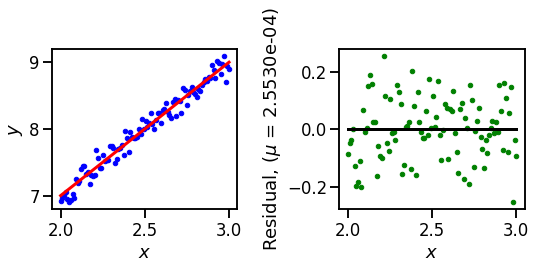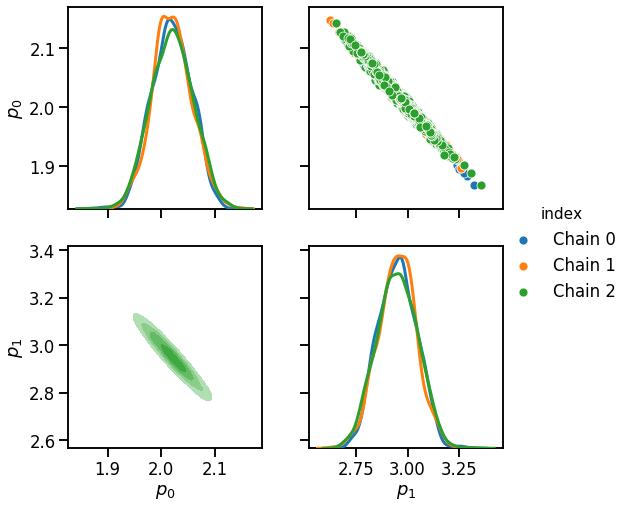# Running Parallel Chains¶

Author(s): Paul Miles | Date Created: August 31, 2018

This demonstration was made using version 1.6.0 of pymcmcstat.

Determining whether or not your chains have converged to the posterior density is a significant challenge when performing MCMC simulations. There are many diagnostics available for assessing chain convergence. The most robust approach is to use the Gelman-Rubin diagnostic, which requires several sets of chains for comparison. The Gelman-Rubin approach essentially performs an analysis of the variances within each chain set and between each chain set. For more details regarding this approach see

While this diagnostic approach does not require computations to be run in parallel, it is certainly more convenient if you can generate a set of chains by running parallel MCMC simulations. In this tutorial we demonstrate how to use the ParallelMCMC class of the pymcmcstat package.

In :
# import required packages
import numpy as np
import os
from pymcmcstat.MCMC import MCMC
from pymcmcstat.ParallelMCMC import ParallelMCMC
from datetime import datetime
import matplotlib.pyplot as plt
import seaborn as sns
import pymcmcstat
print(pymcmcstat.__version__)

1.9.0


# Define Model and Sum-of-Squares Function¶

In :
# define test model function
def modelfun(xdata, theta):
m = theta
b = theta
nrow, ncol = xdata.shape
y = np.zeros([nrow,1])
y[:, 0] = m*xdata.reshape(nrow,) + b
return y

def ssfun(theta, data):
xdata = data.xdata
ydata = data.ydata
# eval model
ymodel = modelfun(xdata, theta)
# calc sos
res = ymodel[:, 0] - ydata[:, 0]
return (res**2).sum(axis = 0)


# Define Data Set - Plot¶

In :
nds = 100
m = 2.0
b = 3.0
x = np.linspace(2, 3, num=nds).reshape(nds, 1)
y = m*x + b + 0.1*np.random.standard_normal(x.shape)
res = y - modelfun(x, [m, b])

sns.set_context('talk')
plt.figure(figsize=(8,4))
plt.subplot(1,2,1)
plt.plot(x, y, '.b');
plt.plot(x, modelfun(x, [m, b]), '-r', linewidth=3)
plt.xlabel('$x$')
plt.ylabel('$y$')
plt.subplot(1,2,2)
plt.plot(x, res, '.g')
mr = res.mean()
plt.plot([x, x[-1]], [mr, mr], '-k', linewidth=3)
plt.xlabel('$x$')
plt.ylabel(str('Residual, ($\\mu$ = {:5.4e})'.format(mr)))
plt.tight_layout(rect=[0, 0.03, 1, 0.95])# Initialize MCMC Object and Setup Simulation¶

• In the simulation options, we turn off the waitbar and set the output verbosity to 0. The results will be saved to a json file.
• We define a directory name based on the date/time to ensure we do not overwrite any results.
• We add the model parameters, but their initial values will actually be determine later.
In :
mcset = MCMC()
datestr = datetime.now().strftime('%Y%m%d_%H%M%S')
savedir = 'resources' + os.sep + str('{}_{}'.format(datestr, 'parallel_chains'))
mcset.simulation_options.define_simulation_options(
savedir=savedir, savesize=1000, save_to_json=True,
verbosity=0, waitbar=False, save_lightly=True, save_to_bin=True)
mcset.model_settings.define_model_settings(sos_function=ssfun)
theta0=2.,
minimum=-10,
maximum=200,
sample=1)
theta0=2.75,
minimum=-10,
maximum=100,
sample=1)


# Setup Parallel Simulation and Define Initial Values¶

• You can specify the number of chains to be generated (num_chain) and the number of cores to use (num_cores).
• Note, the initial values are defined in a 3x2 array. The expected size is [num_chain x num_par], which is 3x2 in this case. If you don't specify initial values, then a random set will be generated within the parameter space.
In :
# setup parallel MCMC
parMC = ParallelMCMC()
initial_values = np.array([[2.5, 2.5], [1.8, 3.8], [2.05, 3.42]])
parMC.setup_parallel_simulation(mcset=mcset,
initial_values=initial_values,
num_chain=3,
num_cores=4)


# Run Parallel Simulation and Display Chain Statistics¶

In :
parMC.run_parallel_simulation()
parMC.display_individual_chain_statistics()

Processing: resources/20190719_131740_parallel_chains/chain_0
Processing: resources/20190719_131740_parallel_chains/chain_1
Processing: resources/20190719_131740_parallel_chains/chain_2
Parallel simulation run time: 1.7680649757385254 sec

****************************************
Displaying results for chain 0
Files: resources/20190719_131740_parallel_chains/chain_0

------------------------------
name      :       mean        std     MC_err        tau     geweke
m         :     2.0206     0.0372     0.0017    13.7387     0.9941
b         :     2.9497     0.0903     0.0042    14.3948     0.9910
------------------------------
==============================
Acceptance rate information
---------------
Results dictionary:
Stage 1: 17.16%
Stage 2: 52.70%
Net    : 69.86% -> 3493/5000
---------------
Chain provided:
Net    : 69.86% -> 3493/5000
---------------
Note, the net acceptance rate from the results dictionary
may be different if you only provided a subset of the chain,
e.g., removed the first part for burnin-in.
------------------------------

****************************************
Displaying results for chain 1
Files: resources/20190719_131740_parallel_chains/chain_1

------------------------------
name      :       mean        std     MC_err        tau     geweke
m         :     2.0180     0.0424     0.0032    22.3207     0.9806
b         :     2.9565     0.1074     0.0081    21.9919     0.9662
------------------------------
==============================
Acceptance rate information
---------------
Results dictionary:
Stage 1: 22.72%
Stage 2: 54.94%
Net    : 77.66% -> 3883/5000
---------------
Chain provided:
Net    : 77.66% -> 3883/5000
---------------
Note, the net acceptance rate from the results dictionary
may be different if you only provided a subset of the chain,
e.g., removed the first part for burnin-in.
------------------------------

****************************************
Displaying results for chain 2
Files: resources/20190719_131740_parallel_chains/chain_2

------------------------------
name      :       mean        std     MC_err        tau     geweke
m         :     2.0158     0.0512     0.0049    40.5945     0.9675
b         :     2.9616     0.1299     0.0127    42.6359     0.9448
------------------------------
==============================
Acceptance rate information
---------------
Results dictionary:
Stage 1: 15.16%
Stage 2: 53.60%
Net    : 68.76% -> 3438/5000
---------------
Chain provided:
Net    : 68.76% -> 3438/5000
---------------
Note, the net acceptance rate from the results dictionary
may be different if you only provided a subset of the chain,
e.g., removed the first part for burnin-in.
------------------------------


# Processing Chains¶

For this example I assume that the MCMC simulations were run, then the user went to process the saved results at a later time. We load the results from the simulation and demonstrate how to plot the parameter distributions and pairwise correlation using the mcmcplot package.

To load the results we must specify the name of the top level directory where the parallel results are stored. We previously defined the savedir variable, so it still exists in memory. If it wasn't, then we would need to define it as the string matching the directory name.

In :
savedir

Out:
'resources/20190719_131740_parallel_chains'
In :
from pymcmcstat import mcmcplot as mcp
from pymcmcstat.chain import ChainProcessing as CP

combined_chains, index = CP.generate_combined_chain_with_index(pres)

settings = dict(
pairgrid=dict(height=3.75, hue='index', despine=False),
ld_type=sns.kdeplot,
md=dict(lw=3))
sns.set_context('talk')
fpg = mcp.plot_paired_density_matrix(
chains=combined_chains,
settings=settings,
index=index)Qualitatively, it appears as though the chains have converged to about the same distribution.

# Gelman-Rubin Diagnostic¶

In the previous section we generated the list of chains which are required for the Gelman-Rubin diagnostic. Note, this implementation assumes that all chains have the same dimension. The output will be a dictionary where the keys are the parameter names. Each parameter references another dictionary which contains

• R, Potential Scale Reduction Factor (PSRF)
• B, Between Sequence Variance
• W, Within Sequence Variance
• V, Mixture-of-Sequences Variance
• neff, Effective Number of Samples
In :
from pymcmcstat.chain import ChainStatistics as CS
chains = CP.generate_chain_list(pres)
psrf = CS.gelman_rubin(chains=chains, display=True)

Parameter: $p_{0}$
R = 0.9999050250834056
B = 0.0005446504072835234
W = 0.0013828493830858575
V = 0.0013825867235498412
neff = 7500
Parameter: $p_{1}$
R = 0.9998927399338449
B = 0.0030520757695050565
W = 0.008775468759398558
V = 0.008773586345638533
neff = 7500


In general, R closer to 1 indicates your chains have converged.